# Bootstrap Simulation with Portfolio Optimizer: Usage for Financial Planning

In statistics, a bootstrap method, also called bootstrapping, is a compute-intensive procedure that allows to estimate the distribution of a statistic through repeated resampling from a single observed sample of data1.

Bootstrapping has several applications in quantitative finance, for example to test the robustness of a trading strategy, to compute a portfolio value at risk, etc.

In this post, I will describe the different bootstrap methods that are implemented in Portfolio Optimizer and I will illustrate the usage of a bootstrap method for financial planning.

Notes:

### Mathematical preliminaries

A bootstrap method typically follows the general three-step Monte Carlo algorithm described below3:

1. Bootstrap simulation step
Generate $k \geq 1$ bootstrap samples $\hat{X}_{1,i}, …, \hat{X}^{*}_{n,i}, i=1..k$ through $k$ independent resamples with replacement from a sample of data $X_1, …, X_n$45 observed from a population

2. Statistic computation step
Compute a statistic of interest on the $k$ generated bootstrap samples

3. Statistic sampling distribution estimation step
Approximate the sampling distribution of the statistic of interest thanks to the computed values of that statistic on the $k$ generated bootstrap samples

In the step 1 above, different resampling schemes lead to different bootstrap methods with different theoretical and practical properties.

In Portfolio Optimizer, three bootstrap methods are supported:

• The IID bootstrap
• The circular block bootstrap
• The stationary block bootstrap

#### Bootstrap method for non-dependent data - IID Bootstrap

The i.i.d. bootstrap method, originally introduced by Efron6, uses as resampling scheme a uniform sampling with replacement.

This bootstrap method is illustrated in Figure 1.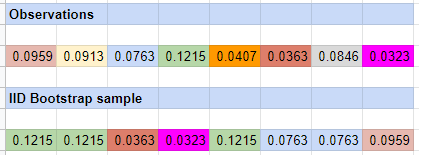Figure 1. Example of i.i.d. bootstrap.

Theoretically, using the i.i.d. bootstrap method for inference requires that the observed sample of data consists of independent and identically distributed (i.i.d.) observations from a population7.

As a consequence, the i.i.d. bootstrap method is not appropriate in case of dependent data8, because resampling the individual observations $X_i, i=1..n$ independently of each other ignores any dependence structure (e.g. serial correlation) present in the observed sample of data.

This issue is illustrated in the context of U.S. equity returns by Capital Spectator in his blog post A Better Way To Run Bootstrap Return Tests: Block Resampling, from which Figure 2 and the associated comment is taken.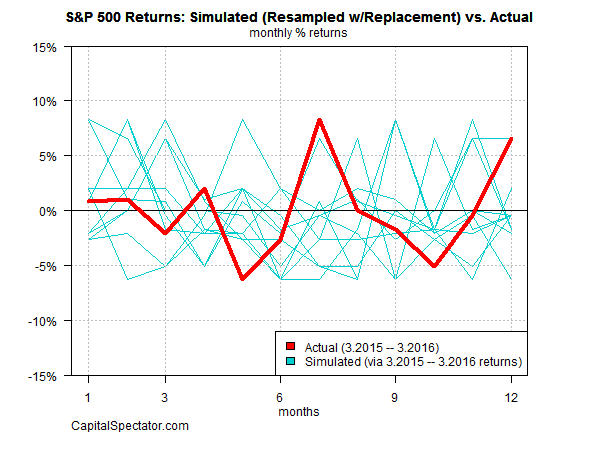Figure 2. Example of i.i.d. bootstrap for the S&P 500 monthly returns. Source: Capital Spectator

The problem is that the tendency for autocorrelation is severed [with the i.i.d. bootstrap]. In other words, the bootstrap sample is too random — the returns are independent from one another. In reality, that’s not an accurate description of market behavior.

#### Bootstrap methods for dependent data

Since the publication of the seminal paper of Efron, the i.i.d. bootstrap method has been extended to the case of dependent data by several authors, including for example Hall9, Carlstein10, Künsch11 and Liu and Singh12, and under several names (non-overlapping block bootstrap, moving block bootstrap…).

The common idea of most of these bootstrap methods for dependent data is to resample with replacement blocks of consecutive observations instead of single observations, which allows the dependence structure present within each block of the observed sample of data to be preserved.

Here again, different block resampling schemes lead to different bootstrap methods for dependent data, with different theoretical and practical properties.

Theoretically, using these block bootstrap methods for inference requires that the observed sample of data consists of weakly dependent stationary observations from a population2.

To be noted, though, that it has been demonstrated that the moving block bootstrap method and the stationary block bootstrap method are both compatible with mild non stationarity13, which encompasses specific forms of serial dependence and heteroscedasticity.

##### Circular Block Bootstrap

The circular block bootstrap method, introduced by Politis and Romano14, uses as resampling scheme a uniform sampling with replacement of circular overlapping blocks of fixed length.

This bootstrap method is illustrated in Figure 3.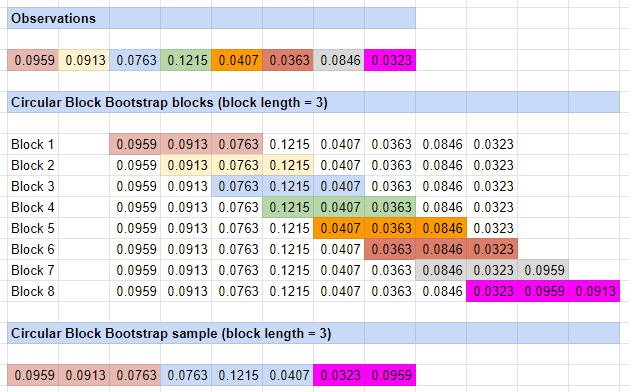Figure 3. Example of circular block bootstrap.

The circular block bootstrap method is an extension of the moving block bootstrap method of Künsch11 and Liu and Singh12 designed to fix the main shortcoming of the latter, which is that the observations at the beginning and at the end of the observed sample of data are undersampled in the bootstrap samples14.

The solution proposed by Politis and Romano visually consists in wrapping the observed sample of data around in a circle7.

##### Stationary Block Bootstrap

The stationary block bootstrap method, also introduced by Politis and Romano15, uses as resampling scheme a uniform sampling with replacement of circular overlapping blocks of random length.

Politis and Romano propose to use a geometric distribution with parameter $p \in ]0,1]$ for the blocks length, with $\frac{1}{p}$ corresponding to the average length of a block, in order to guarantee a theoretical property called conditional stationarity7, but any distribution can be used for the blocks length.

This bootstrap method is illustrated in Figure 4.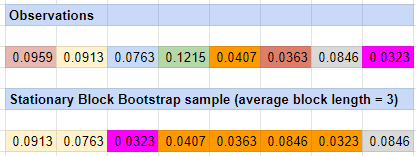Figure 4. Example of stationary block bootstrap.
##### How to choose the block length?

It has been shown2 both theoretically and empirically that the performances of block bootstrap methods depend significantly on the chosen block length16.

In order to choose an appropriate block length, it is possible to refer to:

• Theoretical results on the (asymptotic) optimal block length that have been established for example by Hall et al.17, who showed that the

[…] optimal block size depends significantly on context, being equal to $n^{\frac{1}{3}}$, $n^{\frac{1}{4}}$ and $n^{\frac{1}{5}}$ in the cases of variance or bias estimation, estimation of a one-sided distribution function, and estimation of a two-sided distribution function, respectively

• Practical results on the (finite sample) optimal block length that have been established for example by Politis and White18 and by Patton, Politis and White19, who introduced a data-driven procedure to compute an optimal block length based on the auto-correlation function of the observed sample of data.

In Portfolio Optimizer, the default block length (resp. average block length) has been chosen to be the same as in the R package tseries, that is, $\lfloor 3.15 n^{\frac{1}{3}} \rfloor$ (resp. $3.15 n^{\frac{1}{3}}$), where $n$ is the size of the observed sample of data.

#### When the bootstrap method fails

Politis et al.20 note that

Bootstrap methods can often achieve the goal of working in complex situations without imposing unrealistic or unverifiable assumptions about the data-generating mechanism, but like any statistical method, they cannot be applied without thought […] and [they] sometimes can fail.

Below are two examples of situations in which bootstrap methods can fail.

First, bootstrap methods are fundamentally dependent on the quality of the observed sample of data.

If this sample is not a reasonable representation of the underlying population (too small sample, biased sample, sample with an incorrect dependence structure…), the sampling distribution of any statistic of interest computed on the bootstrap samples will not be accurate.

Second, bootstrap methods are known to be inconsistent in some specific cases.

For example21, in the case of the i.i.d. bootstrap:

• The sampling distribution of the maximum of random variables converges to a random c.d.f.
• The sampling distribution of the mean of heavy tailed random variables converges to a random c.d.f.

Fortunately, it is usually possible to fix the inconsistency of a bootstrap method by generating bootstrap samples of $m < n$ observations22, but it is difficult in practice to determine a priori whether a given bootstrap method will be inconsistent for a given sample of observed data and a given statistic of interest.

As a side note, Portfolio Optimizer allows to generate bootstrap samples of any number of observations.

### Example of usage - Financial planning

Since the seminal paper Determining Withdrawal Rates Using Historical Data of Bengen23, financial planning using computer-based simulations, also known as Monte Carlo simulations, has become standard practice, c.f. for example the blog post The 3rd Generation of Financial Planning from Tolerisk or the sheer number of software providers offering goal based investing software solutions24.

Although the future is impossible to predict, such simulations help to set realistic expectations about the potential performances of financial portfolios over long horizons and under real-life constraints25 (e.g., periodic contributions or withdrawals, taxes, rising inflation rate, exposure to a sequence of return risk due to a bear market occurring at the wrong time…).

As an illustration of how Portfolio Optimizer could be integrated into a financial planning software, I will use the methodology of Anarkulova et al.26 to simulate how a portfolio of U.S. stocks could be expected to behave over the next 30 years.

The exact methodology is the following:

1. Use the stationary block bootstrap method with an average block length of 120 months27 to generate 10,000 bootstrap samples of 360 monthly returns28 $\hat{R}_{1,i}, …, \hat{R}_{360,i}, i=1..10,000$ from the 1,428 U.S. stock market monthly returns28 over the period January 1871 - December 1989 collected by Robert J. Shiller on his website
2. For each bootstrap sample $i=1..10,000$ and for each $h$-month horizon $h=1..360$, compute the projected cumulative wealth29 at the $h$-month horizon $W_{h,i}$ associated to a fully invested buy and hold portfolio of U.S. stocks, with $W_{h,i}$ defined by $W_{h,i} = \prod_{t=1}^{h} \left( 1 + \hat{R}_{t,i} \right)$
3. For each $h$-month horizon $h=1..360$, compute the sampling distribution of $W_{h,i}$ over all the 10,000 bootstrap samples, and in particular compute
• The median of this sampling distribution, which represents the (robust) expected projected cumulative wealth at the $h$-month horizon
• The 2.5-th and 97.5-th percentiles of this sampling distribution, which represent the 95% bootstrap percentile confidence interval3 of the projected cumulative wealth at the $h$-month horizon
• The 12.5-th and 87.5-th percentiles of this sampling distribution, which represent the 75% bootstrap percentile confidence interval3 of the projected cumulative wealth at the $h$-month horizon

More on why I considered U.S. stock returns only up to December 1989 later.

Step 1 is easily implemented thanks to the Portfolio Optimizer endpoint /assets/returns/simulation/bootstrap, while the ease of implementation of the remaining steps depends heavily on the environment (Google Sheets, C/C++, Python…)30.

The results of step 2, that is, 10,000 projected cumulative wealth paths over 360 months, are illustrated in Figure 5.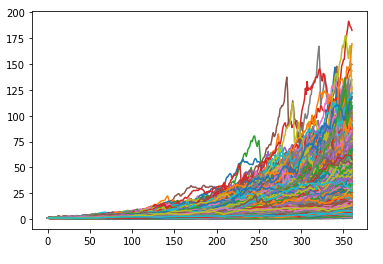Figure 5. Example of Monte Carlo simulation of asset returns (bootstrap) with Portfolio Optimizer.

For the sake of comparison, the results of an arbitrary Monte Carlo simulation performed by the Moneytree financial planning solution, taken from a presentation of Moneytree, are illustrated in Figure 6.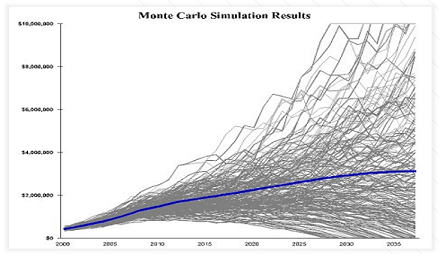Figure 6. Example of Monte Carlo simulation with Moneytree. Source: Moneytree.

The similarities are striking…

The results of the step 3, that is, 360 medians and 360 2.5-th/12.5-th/87.5-th/97.5-th percentiles, are illustrated in Figure 7, together with the realized cumulative wealth over the period January 1990 - December 2019 which corresponds to the U.S. stock returns that I held out on purpose.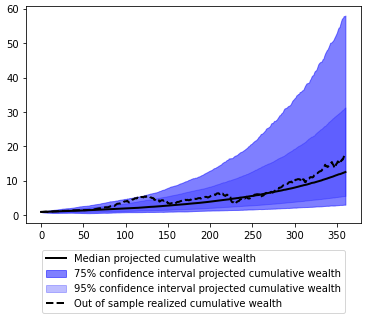Figure 7. Example of Monte Carlo simulation of asset returns (bootstrap) with Portfolio Optimizer, and comparison with realized U.S. stock returns.

What is now striking is that the realized U.S. stock returns over the held-out period January 1990 - December 2019 are nearly always contained within the 95% bootstrap percentile confidence interval and are around three-quarter of the time contained within the 75% bootstrap percentile confidence interval…

While it seems that there might be black magic at work, this gives serious credibility to Monte Carlo simulations for financial planning!

### Conclusion

Although this post only scratched the surface of the usage of bootstrap methods in portfolio management, I hope this feature of Portfolio Optimizer will be useful!

1. A strong assumption in any bootstrap method is that the observed sample of data is a reasonable representation of the underlying population, which somewhat guarantees that the distribution of the statistic over the resamples is a good approximation of the distribution of the statistic over the whole population.

2. The observations $X_1, …, X_n$ can be random variables or random vectors.

3. In some bootstrap methods, like the parametric bootstrap, the observations $X_1, …, X_n$ are first used to build a statistical model of the population from which the bootstrap samples are then generated.

4. Hence the name of this bootstrap method.  2 3

5. There are different notions of dependent data in the literature, but the i.i.d. bootstrap is already not appropriate when the observed sample of data consists of $p$-dependent observations from a population, $p \geq 1$, c.f. Lahiri2

6. Whether a fixed block length (moving block bootstrap, circular block bootstrap…) or an average block length (stationary bootstrap).

7. This tweak is called the “$m$ out of $n$ bootstrap”21, and leads to the additional question of how to best choose the parameter $m$…

8. Goal based investing software solutions, for example developed by MoneyGuidePro or WealthTrace, are typically using computer-based simulations due to the complexity of the underlying mathematical models.

9. Such constraints are particularly important to take into account in the context of retirement planning, where the horizon is sometimes 50+ years.

10. Such an average block length has been shown26 to capture the stylized facts of asset returns, including time-varying volatility and mean reversion.

11. The monthly returns are total nominal returns (i.e., the consumer price index was forced to 1 in Shiller’s Excel sheet to explicitly discard inflation).  2

12. Starting from 1\$.

13. I used Python, c.f. the Jupyter notebook corresponding to this post available on Binder -Tags:

Updated: